# Electric Circuits Examples

By | March 28, 2023

Electric Circuits Examples: Electricity Powers the World

We use electricity every day, but it's not something we typically think about. From the lights in our homes to the computers that power our businesses, electricity is everywhere and it powers the world around us. But do you know how it works? Electric circuits are the basis of nearly all electronic devices. Understanding the basic components of a circuit, and how they work together, can help you make sense of the technology that surrounds us.

An electric circuit is a closed loop of conductive material, through which an electrical current flows. The circuit is completed when the two ends of the conductive material are connected. This connection is known as the load, and it is where the electricity is used. The load is typically a device such as a light bulb or a motor. For our purposes, we will be discussing basic examples of electric circuits.

The simplest electric circuit is a series circuit, which consists of one wire connected to a switch and a source of voltage (such as a battery). When the switch is closed, electricity flows from the battery, through the switch, and back to the battery again, completing the circuit. In a parallel circuit, multiple wires are connected to the same switch, allowing the current to flow through each wire separately. This allows for multiple loads to be powered from the same circuit.

A common example of both series and parallel circuits is a holiday light display. In a series circuit, each strand of lights is connected to the next, so that if one strand fails, the entire display stops working. In a parallel circuit, each strand is connected independently, so that if one strand fails, the rest of the display remains lit.

Electronics are more complex than simple series and parallel circuits. To understand how more complex circuits operate, it's important to understand the components which make them up. Some of the most common components in circuits include resistors, capacitors, and transistors. Resistors restrict the flow of electricity through a particular path, while capacitors store and release electrical energy, and transistors act as switches, controlling the flow of current in a circuit. Other components such as diodes and inductors are also used in more complex circuit designs.

Electronic circuits, from the simplest Christmas lights to the most complex computers, all rely on the same basic principles. Understanding these concepts and how they interact with each other can help you better understand the technology that surrounds us. We hope this overview has given you a better understanding of electric circuits examples and the role they play in powering the world around us.Electrical Circuits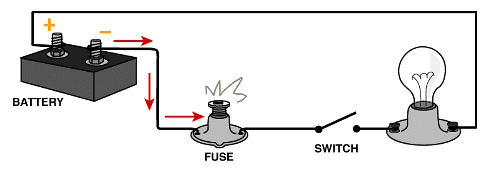Fundamentals Of Electricity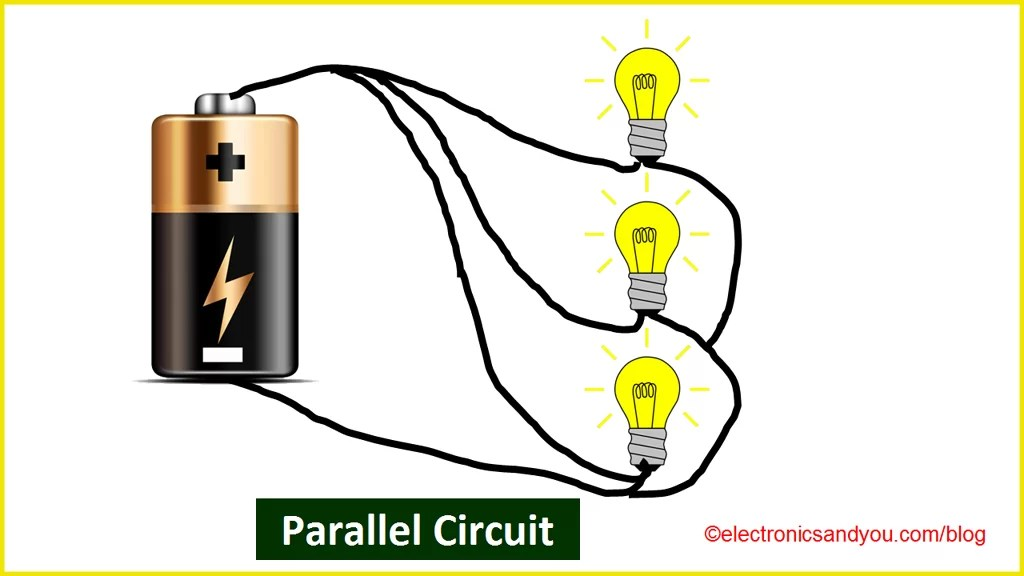Types Of Electric Circuit Definition Examples SymbolsElectrical Symbols Circuit Diode Logic Gates FaqsClosed Open And Short Circuits DummiesParts Of A Circuit Lesson For Kids Transcript Study ComSolved Give Five Examples Of Situations Or Applications Where Electronic Circuits And Electric Are TogetherA Few Examples Of The Electric Circuits Depicted In Experiment Scientific DiagramTypical Example Of An Electric Circuit Scientific DiagramSimple Electric Circuit Royalty Free Vector ImageSeries And Parallel Dc Circuits Explained Examples Included Electrical4uElectrical Circuits For Kids Circuit Types Dk Find OutElectric Circuits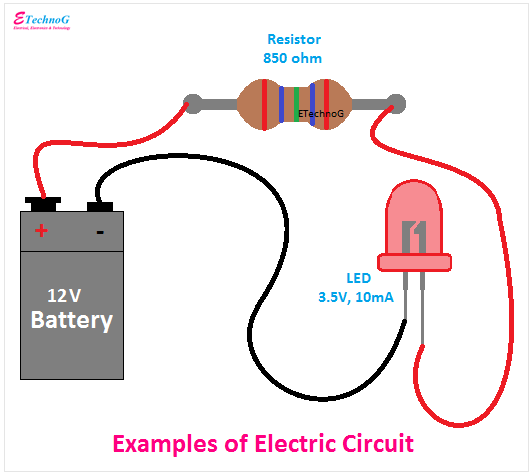What Is Electric Circuit Examples Types Components EtechnogExamples Of Neuronal Circuits A Electrical Circuit The Hodgkin Scientific Diagram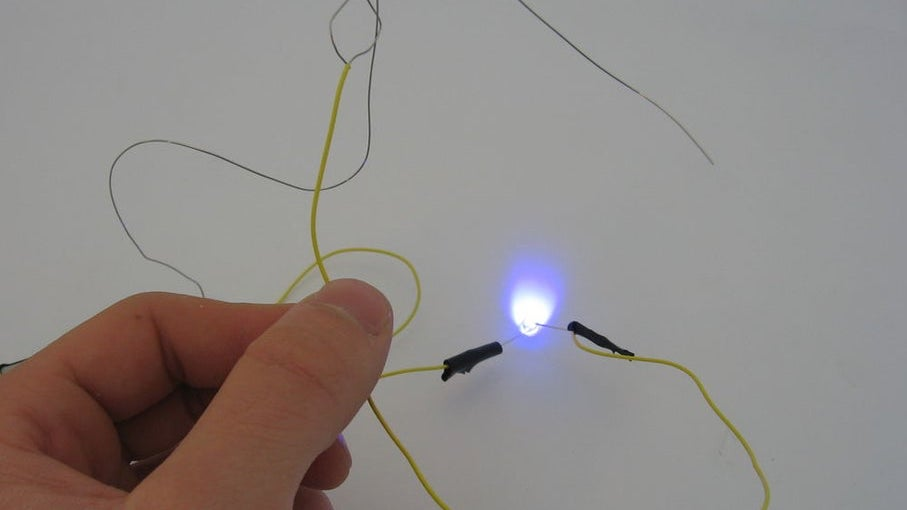7 Exciting Electric Circuit Projects For Kids Stem Education GuideMathonweb Analysing Electric Circuits And Networks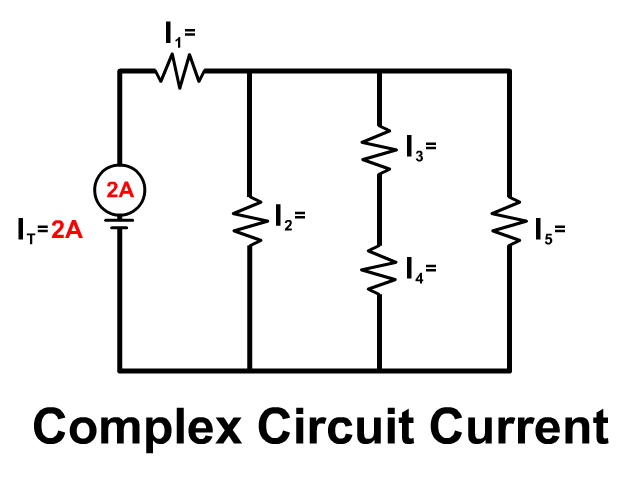Complex Circuit Stickman Physics